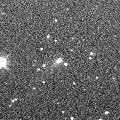# \$B%j%K%"WB@1(B

C/1999 S3 ( LINEAR )

 English version Home page Updated on November 11, 2005###\$B%W%m%U%#!<%k(B

 \$BH/8+F|(B 1999\$BG/(B 9\$B7n(B24\$BF|(B \$BH/8+8wEY(B 15.7\$BEy(B \$BH/8+ Lincoln Laboratory Near-Earth Asteroid Research project

###\$B###\$B50F;MWAG(B

```   The following improved orbital elements by Kenji Muraoka, are
from 616 observations 1999 Sept. 24 to 2000 Feb. 29, perturbations
by 9 Planets, Moon and 5 minor planets were taken into account.
The mean residual is +/- 0.57 arc seconds.

Epoch  =  1999 Oct. 29.0  TT       JDT = 2451480.5
T  =  1999 Nov.  9.01313       +/- 0.00068 (m.e.) TT
Peri. =   44.12690                +/- 0.00030
Node  =   11.87847                +/- 0.00015   (2000.0)
Incl. =   70.56147                +/- 0.00014
q  =    1.8949606              +/- 0.0003042 AU
e  =    0.8999951              +/- 0.0000112
a  =   18.9486696              +/- 0.0021711 AU
n  =    0.01194912             +/- 0.00000205
P  =   82.484                  +/- 0.0141763  years
(+/- 5.2 days)
```

###\$B@1?^(B###\$B8wEYJQ2=(B

```        m1 = -5.5 + 5 log\$B&\$(B + 60 log r(t - 15)
```##### \$B50F;MWAG\$OB<2,7r<#;a\$N7W;;\$K\$h\$k\$b\$N\$G\$9!#(B \$B@1?^\$O(B StellaNavigator Ver.2.0 for Windows (\$B%"%9%H%m%"!<%D(B \$BJTCx(B / \$B%"%9%-!<=PHG6I4)(B) \$B\$G:n@.\$7\$?\$b\$N\$G\$9!#(B \$B8wEY%0%i%U\$O(BComet for Windows\$B\$G:n@.\$7\$?\$b\$N\$G\$9!#(B